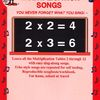# 8x2 to 8x12 from "Multiplication Songs" by Kathy Troxel/Audio Memory

Memorize the "8x2 to 8x12" Song from "Multiplication Songs" by Kathy Troxel/Audio Memory.
Practice with the "8x2 to 8x12 Song Test" (facts in order).
Take the KwizMi test (facts out of order).

• Fill in the blanks.
• 8 x11 =
•
• 8 x 9 =
•
• 8 x 4 =
•
• 8 x 12 =
•
• 8 x 5 =
•
• 8 x 3 =
•
• Fill in the blanks.
• 8 x 7 =
•
• 8 x 2 =
•
• 8 x 10 =
•
• 8 x 1 =
•
• 8 x 6 =
•
• 8 x 8 =
•

### Tags

Multiplication tables, Multiplication songs, Times tables, Timed test, Multiplication facts, 8 times table